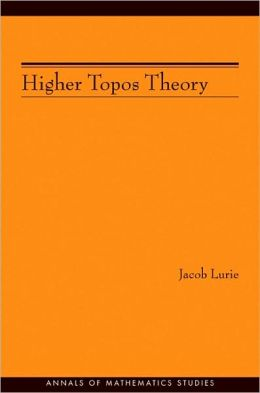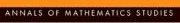## Series Monográficas: Annals of Mathematics StudiesVol. 21 Von Neumann, John
Functional operators
QA320 V64 V.1

Vol. 10 Lefschetz, Solomon
Topics in topology
QA611 L418

Vol. 20 Lefschetz, Solomon,  (ed.)
Contributions to the theory of nonlinear oscillations
QA871 L44

Vol. 9 Sewell, Walter Edwin
Degree of approximation by polynomials in the complex domain
QA281 S47

Vol. 19 Bochner, Salomon; Chandrasekharan, Komaravolu
Fourier transforms
QA404 B63

Vol. 8 Busemann, Herbert
Metric methods in finsler spaces and in the foundations of geometry
QA680 B86

Vol. 18 Truesdell, Clifford
An essay toward a unfield theory of special functions based upon the functional equation 8/ 8z f z,a - f z,a+1
QA431 T77

Vol. 7 Halmos, Paul  Richard
Finite-dimensional vector spaces
QA261 H32

Vol. 17 Liapunov, Aleksandr Mikhailovich
Probleme general de la stabilite du mouvement
QA871 L75

Vol. 6 Church, Alonzo
The calculi of lambda-conversion
QA331 C52

Vol. 16 Siegel, Carl Ludwing
Transcedental numbers
QA247.5 S5 1949

Vol.  5 Post, Emil Leon
The two-valued iterative systems of mathematical logic
QA9 P8

Vol. 15 Morse, Marston
Topological methods in the theory of functions of a complex variable
QA331 M794

Vol.  4  Murray, Francis Joseph
An introduction to linear transformation in hilbert space. Linear transformations in hilbert space
QA601 M86

Vol. 14 Lefschetz, Solomon
Lectures on differential equations
QA371 L38

Vol. 3 Godel, Kurt
The consistency of the axiom of chioce and of the generalized continuu-hypothesis with the axioms of set theory
QA9 G6

Vol. 13 Church, Alonzo
Introduction to mathematical logic
BC135 C42 1944

Vol. 2 Tukey, John Wilder
Convergence and uniformity in topology
QA611 T8

Vol. 12 Weyl, Hermann
Meromorphic functions and analytic curves
QA331 W48

Vol. 1 Weyl, Hermann
Algebraic theory of numbers
QA241 W49

Vol. 11 Kryloff, Nikolai Mitrofanovich
Introduction to non-linear mechanics
QA871 K73

### ¿Quién está en línea?

Hay 402 invitados y ningún miembro en línea

Go to top